## Customer Attrition Model using Decision Tree

By: Ram on Apr 03, 2021

Business Context and Customer Attrition

It is costly to acquire a new customer, hence banks and other organizations work to keep their customer engaged (or reduce the customer attrition)

Customer Attrition can be silent (when a customer stops using a product) and explicit when a customer closes a product.

Typically, there is a journey or path to customer attrition and the organization should be proactive both from identifying the customer progression to this path and taking suitable actions (interventions)

Reduce Engagement -> Dormant/Inactive -> Close Relationship

Identifying early helps in reducing attrition and also with less cost.

In addition, the customer attrition can help identify:

• Issues in Services or Product Features
• Right Strategic Choices to Increase Customer Satisfaction and Engagement

Objective

The objective of this blog is to build a customer attrition model for a bank to identify the customers who have higher chances of attrition.

Steps

• Read Data
• EDA
• Balancing of Data- Under-sampling or Over-Sampling
• Classification Model using Decision Tree
• Parameter Tuning
• Tree Visualization
• Validation

### Read Data

`from google.colab import drive`

`drive.mount('/content/drive')`

`import pandas as pd`

`import zipfile`

`# Create reference to zipped file/folder`

`mr = zipfile.ZipFile('/content/drive/MyDrive//Training/ML - Mar2021/Data/Predicting Churn for Bank Customers.zip')`

`attrition = pd.read_csv(mr.open('Churn_Modelling.csv'))`

View a few observations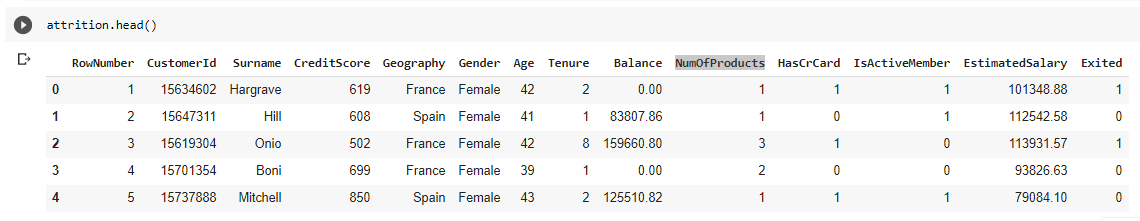DataFrame Information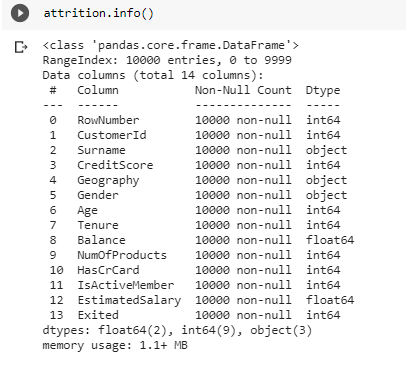The data frame has 14 columns and 10K customers. And the last column is Label Feature

Target Variable Distribution

Target Variable: Exited

We can check the distribution and the value being coded.

• Exited = 0, for non-churned customers
• Exited = 1, for churned customers

`import warnings`

`warnings.simplefilter(action = 'ignore', category = FutureWarning)`

`import matplotlib.pyplot as plt`

`import seaborn as sns`

`colors = ['Green', 'Orange']`

`fig = plt.figure(figsize = (6, 4))`

`sns.countplot(x = 'Exited', data = attrition, palette = colors)`

`for index, value in enumerate(attrition['Exited'].value_counts()):`

`    label =  '{}%'.format(round( (value/attrition['Exited'].shape)*100, 2)) `

`    plt.annotate(label, xy = (index - 0.18, value - 800), color = 'w', fontweight = 'bold', size = 14)`

`plt.title('Distribution of Customers')`

`plt.xticks([0, 1], ['Still Customer', 'Attrited'])`

`plt.xlabel('Customer Status')`

`plt.ylabel('Customer Count');`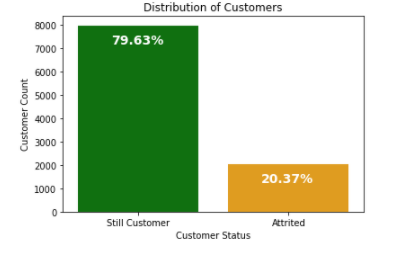If we look at the distribution around 80% of the customers are non-attrition and 20% are attrition.

This is a scenario of imbalanced data. In such a scenario, accuracy is probably not the best metric to measure model performance.

We should make it balance data. There are multiple options such as

Oversampling: Pick up all instance of majority class and then a similar number of instances selected randomly from minority class with selecting with replacement

Undersampling: Pick up all instance of minority class and then a similar number of instances selected randomly from the majority class

Let's go with Undersampling for now.

`import pandas as pd `

`from random import sample `

`# Split the samples based on class`

`attrition_0 = attrition[attrition.Exited==0]`

`attrition_1 = attrition[attrition.Exited==1]`

`# random sampling majority class`

`attrition_0=attrition_0.reset_index()`

`sample_index = sample(range(len(attrition_0)), len(attrition_1))`

`attrition_0_sample = attrition_0.iloc[sample_index]`

`print("No of rows after sampleing", len(attrition_0_sample))`

`attrition_us = pd.concat([attrition_1,attrition_0_sample])`

`import warnings`

`warnings.simplefilter(action = 'ignore', category = FutureWarning)`

`import matplotlib.pyplot as plt`

`import seaborn as sns`

`colors = ['Green', 'Orange']`

`fig = plt.figure(figsize = (6, 4))`

`sns.countplot(x = 'Exited', data = attrition_us, palette = colors)`

`for index, value in enumerate(attrition_us['Exited'].value_counts()):`

`    label =  '{}%'.format(round( (value/attrition_us['Exited'].shape)*100, 2)) `

`    plt.annotate(label, xy = (index - 0.18, value - 800), color = 'w', fontweight = 'bold', size = 14)`

`plt.title('Distribution of Customers')`

`plt.xticks([0, 1], ['Still Customer', 'Attrited'])`

`plt.xlabel('Customer Status')`

`plt.ylabel('Customer Count');`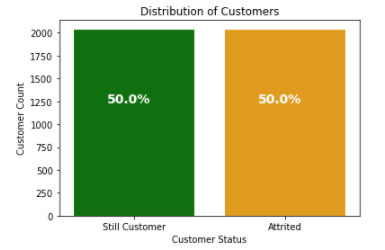### Exploratory Data Analysis - EDA

The target variable is a Binary Variable and we want to understand the relationship of each of the features with the target variables.

• Bivariate – Categorical – Categorical
• Bivariate – Categorical vs Continuous

`def BiVarContPlot(df, depVar, feature):  `

`  import matplotlib.pyplot as plt`

`  import seaborn as sns`

`  colors = ['Green', 'Orange']`

`  df_1 =  df[df[depVar]==1]`

`  df_0 =  df[df[depVar]==0]`

`  fig, (ax1, ax2) = plt.subplots(1, 2, figsize = (12, 4))    `

`  sns.distplot(df_0[feature], bins = 15, color = colors, label = 'Not Attrited', hist_kws = dict(edgecolor = 'firebrick', linewidth = 1), ax = ax1, kde = False)`

`  sns.distplot(df_1[feature], bins = 15, color = colors, label = 'Attrited', hist_kws = dict(edgecolor = 'firebrick', linewidth = 1), ax = ax1, kde = False)`

`  ax1.set_title('{} distribution - Histogram'.format(feature))`

`  ax1.set_ylabel('Counts')`

`  ax1.legend()`

`  sns.boxplot(x = 'Exited', y = feature, data = df, palette = colors, ax = ax2)`

`  ax2.set_title('{} distribution - Box plot'.format(feature))`

`  ax2.set_xlabel('Status')`

`  ax2.set_xticklabels(['Non Attrited', 'Attrited'])`

`  plt.tight_layout();`

`def BiVarCatPlot(df, depVar, feature):`

`    import warnings`

`    warnings.simplefilter(action = 'ignore', category = FutureWarning)`

`    import matplotlib.pyplot as plt`

`    import seaborn as sns`

`    colors = ['Green', 'Orange']`

`    fig, (ax1, ax2) = plt.subplots(1, 2, figsize = (12, 4))`

`    sns.countplot(x = feature, hue = depVar, data = df, palette = colors, ax = ax1)`

`    ax1.set_ylabel('Counts')`

`    ax1.legend(labels = ['Non Attrited', 'Attrited'])`

`    colors2 = sns.color_palette("husl", len(df[feature].value_counts()))`

`    sns.barplot(x = feature, y = depVar, data = df, palette = colors2 , ci = None, ax = ax2)`

`    ax2.set_ylabel('Churn rate')   `

`    plt.tight_layout();`

#### Age

Hypothesis: Age may play a role in customer attrition. Younger customers may have higher attrition.

We can see % attrition across Age Buckets. A histogram can be helpful in checking the distributions for each of the attrition and non-attrition groups.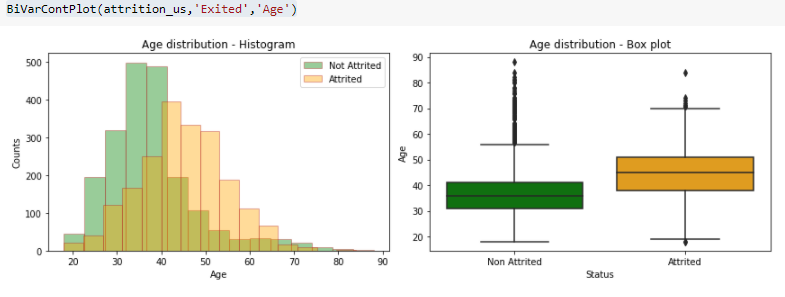Summary Statistics

`attrition_us.groupby('Exited').agg({'Age': ['mean', 'count']})`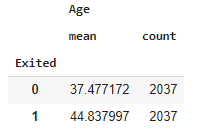It is quite evident that age distribution for attrition and non-attrition segments are quite different. Avg age for the attrition is 44 as compared to 37 for the non-attrition segment. So, higher-aged customers are more likely to attrite.

#### Credit Score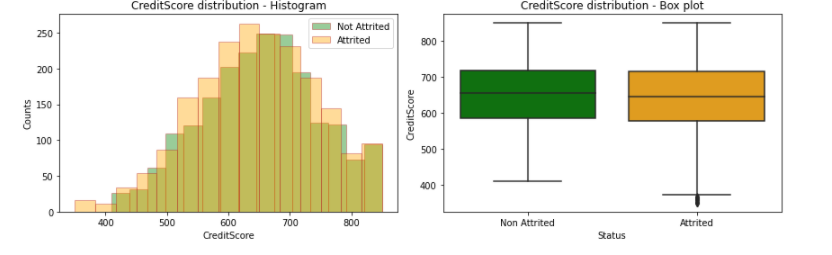It seems from the above charts that there is not much difference in credit score for both the segments (attrition and non-attrition)

#### Balance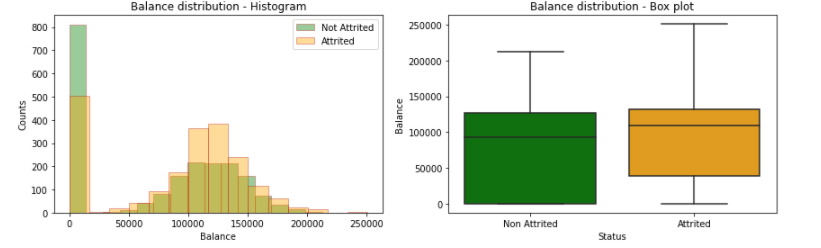There is a high % of customers with 0 balance, we can do further analysis to see if we should create an indicator feature for the customers with 0 or very low balance

Attrition customers seem to have slightly higher balances.

#### Estimated Salary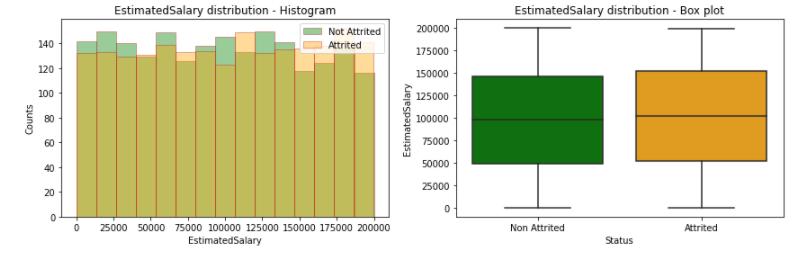It is clear that there is not much of the difference in the distribution and average estimated salary across these 2 segments.

### Categorical Features

#### Geography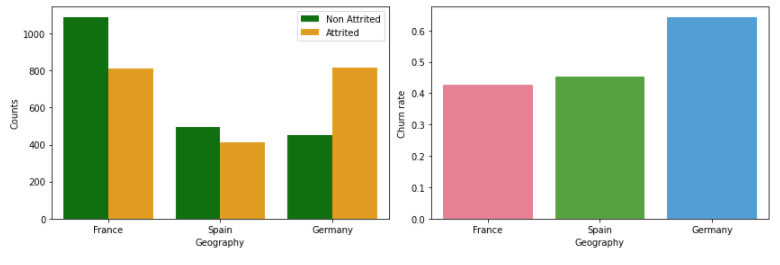Attrition is for France and Spain

#### Gender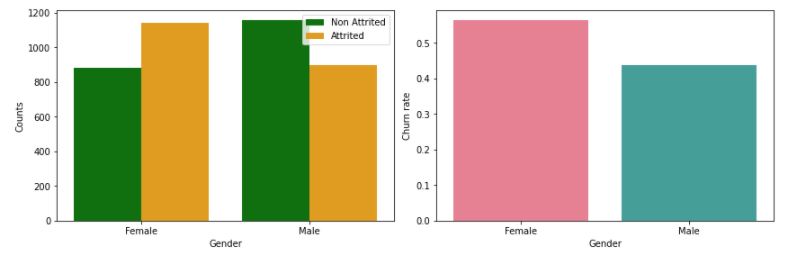Females have a higher representation in attrition.

#### Tenure

Tenure of the customers with the bank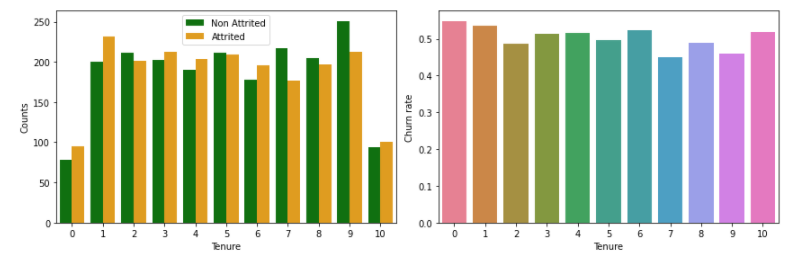Not a strong relationship

#### Number of Products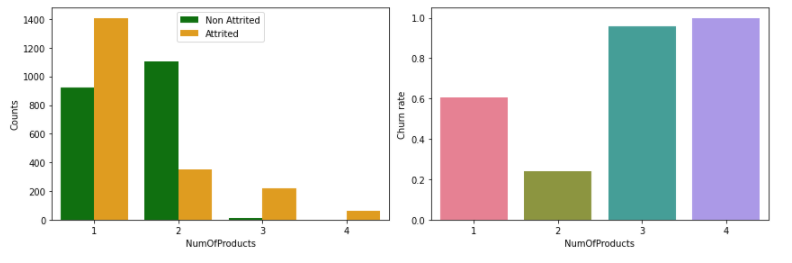This is an interesting scenario. We should combine the above two charts to conclude.

We can create a separate group after combining Numbers of Products 3 and 4 as these groups have small counts.

Other points are that we can see very low attrition for the customers with 2 products, should we combine customers with products 3 and 4 holders with 2?

#### Has Credit Card?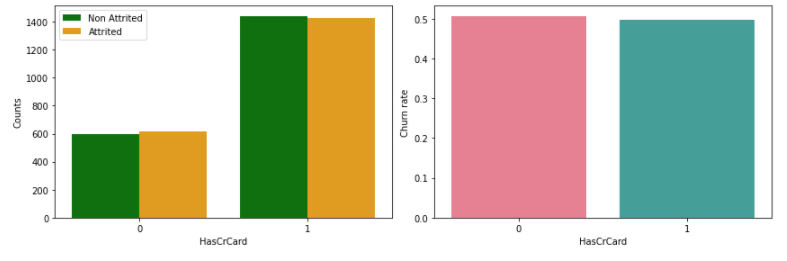Not much different for each of these groups.

#### Is active member?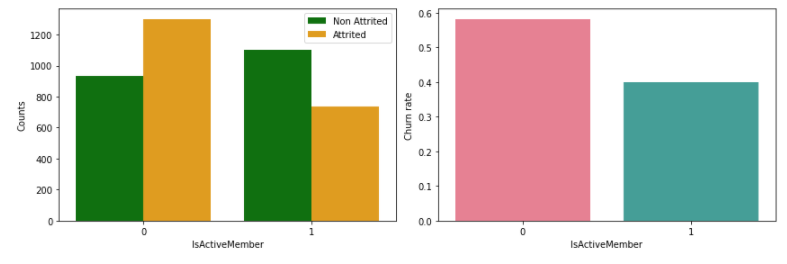Not active members have higher attritions.

### Feature Engineering

Attrition for France and Spain is similar so we can combine and put in the same group. Also, we can create a dummy variable for the geography.

Also, gender is encoded as 1 and 0

`from sklearn import preprocessing`

`attrition_us['Gender'] = preprocessing.LabelEncoder().fit_transform(attrition_us['Gender'])`

`attrition_us['Geography'] = attrition_us['Geography'].map({'Germany': 1, 'Spain': 0, 'France': 0})`

We can also update the groups for NumOfProducts

`import numpy as np`

`attrition_us['NumOfProducts_1plus'] = np.where(attrition_us['NumOfProducts']>=2,1,0)`

`attrition_us['NumOfProducts_1plus'].value_counts()`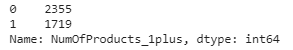### Model Samples

`import numpy as np `

`label =attrition_us['Exited']`

`features = attrition_us.drop(["Exited","Surname","index", 'RowNumber',  'CustomerId','NumOfProducts'], axis=1)`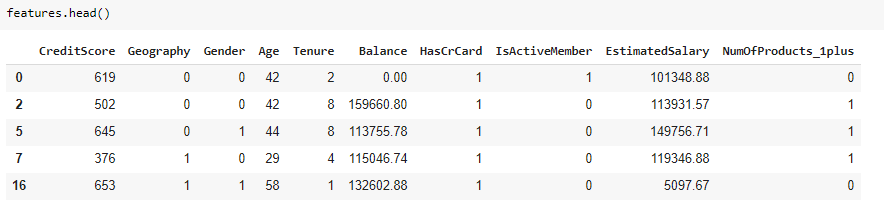`# Split the data into Dev/Train and Val/Test`

`from sklearn.model_selection import train_test_split`

`train_X,test_X,train_Y,test_Y=train_test_split(features,label,test_size=0.2,random_state=0)`

### Decision Tree - CART

`# Load library`

`from sklearn import tree`

`from sklearn.tree import DecisionTreeClassifier`

`# Decision Tree - CART Algorithm (gini criteria): Set up`

`dt_train_gini = DecisionTreeClassifier(criterion = "gini", random_state = 100,`

`                               max_depth=10, min_samples_leaf=200,min_samples_split=500)`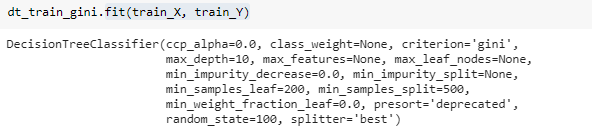### Decision Tree Visualization

`from sklearn.tree import export_graphviz`

`# Export Decision Tree as dot file`

`export_graphviz(dt_train_gini, out_file='/content/drive/My Drive/Training/IIITDM/Output/bank_customer_attrition_tree.dot', `

`                feature_names=train_X.columns,`

`                class_names='Class',`

`                rounded = True, proportion = False, `

`                precision = 2, filled = True)`

`# Convert to png`

`from subprocess import call`

`call(['dot', '-Tpng', '/content/drive/My Drive/Training/IIITDM/Output/bank_customer_attrition_tree.dot', '-o',`

`      '/content/drive/My Drive/Training/IIITDM/Output/bank_customer_attrition_tree.png', '-Gdpi=600'])`

`# Display in python`

`import matplotlib.pyplot as plt`

`plt.figure(figsize = (14, 18))`

`plt.imshow(plt.imread('/content/drive/My Drive/Training/IIITDM/Output/bank_customer_attrition_tree.png'))`

`plt.axis('off');`

`plt.show();`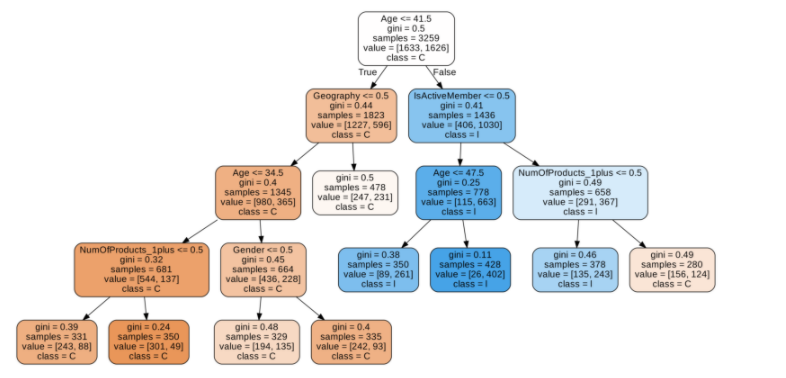### Decision Tree - Hyper Parameter Tuning

There are a number of parameters that can be optimized to select the best combinations. We will try only a few.

`# Load library`

`from sklearn import tree`

`from sklearn.tree import DecisionTreeClassifier`

`for algo in criterion:`

`  for depth in max_depth:`

`    for leafsize in min_leaf:`

`      dt_train_gini = DecisionTreeClassifier(criterion = algo, random_state = 100,`

`                               max_depth=depth,`

`                               min_samples_leaf=leafsize,min_samples_split=500)`

`      dt_train_gini.fit(train_X, train_Y)`

`      # Check Performance`

`      print("Criteria:",algo, "\n Depth: ",depth,"\n Leaf Size", leafsize)`

`      print("Precision",precision_score(train_Y, dt_train_gini.predict(train_X))*100)`

`      print("Recall",recall_score(train_Y, dt_train_gini.predict(train_X))*100)`

We can also use GridSearch to select the optimal parameters.

`from sklearn import tree`

`from sklearn.model_selection import GridSearchCV`

`attrition_tree = tree.DecisionTreeClassifier()`

`# Search Optmizal Paramters`

`attrition_GS = GridSearchCV(attrition_tree, `

`                            param_grid= parameters,`

`                            scoring =scoring_metric,`

`                            cv=10,`

`                            n_jobs=-1)`

`attrition_GS.fit(train_X, train_Y)`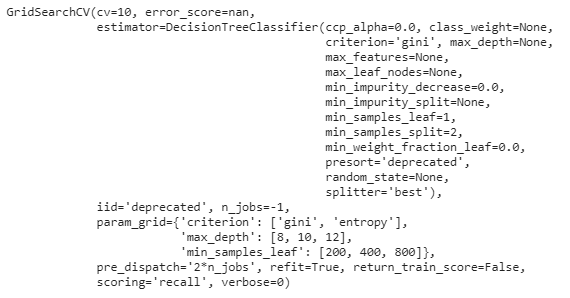`print('Best Criterion:', attrition_GS.best_estimator_.get_params()['criterion'])`

`print('Best max_depth:', attrition_GS.best_estimator_.get_params()['max_depth'])`

`print('Best Min Leaf Node:', attrition_GS.best_estimator_.get_params()['min_samples_leaf'])`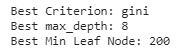`attrition_GS.best_params_`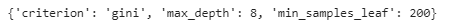### Prediction and Evaluation

`#Calculate the accuracy`

`from sklearn.metrics import accuracy_score`

`from sklearn.metrics import precision_score`

`from sklearn.metrics import recall_score`

`print("Accuracy",accuracy_score(train_Y, dt_train_gini.predict(train_X), normalize=True)*100)`

`print("Precision",precision_score(train_Y, dt_train_gini.predict(train_X))*100)`

`print("Recall",recall_score(train_Y, dt_train_gini.predict(train_X))*100)`

### Performance on Test Sample

`#Calculate the accuracy`

`from sklearn.metrics import accuracy_score`

`from sklearn.metrics import precision_score`

`from sklearn.metrics import recall_score`

`print("Accuracy",accuracy_score(test_Y, dt_train_gini.predict(test_X), normalize=True)*100)`

`print("Precision",precision_score(test_Y, dt_train_gini.predict(test_X))*100)`

`print("Recall",recall_score(test_Y, dt_train_gini.predict(test_X))*100)`

###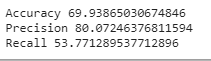Tags
Most PopularJun 18, 2020Jul 23, 2020Jun 19, 2020Jul 05, 2020